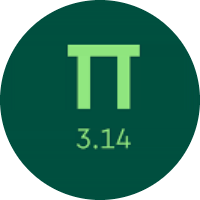# Understanding the Basics of Functions

In this quiz, you will get to know about the basics of functions. You will learn about identifying the functions, linear functions, writing a linear function, and evaluating the linear functions.

Start Quiz

Look at this set of ordered pairs:

(7, 9)
(10, 8)
(18, 5)
(11, 4)

Is this relation a function?

yes

no

Is (0, 3) a solution to the equation y = 4x?

yes

no

Does the point (3, 3) satisfy the equation y = x?

yes

no

Lila and Cora are going on a road trip together. They have a limited budget, so they consider several different routes and calculate the cost of gas for each route. The cost of gas for each route depends on the length of the route.

g = the cost of gas
r = the length of the route

Which of the variables is independent and which is dependent?

r is the independent variable and g is the dependent variable

g is the independent variable and r is the dependent variable

Bruce runs the Harrison Farm, which produces many different crops, including wheat. The number of bushels of wheat produced by the farm each year depends on the number of plots of land that are devoted to growing wheat.

w = the number of bushels of wheat produced each year
p = the number of plots of land devoted to growing wheat

Which of the variables is independent and which is dependent?

w is the independent variable and p is the dependent variable

p is the independent variable and w is the dependent variable

Use the function y = j + 3 to find the value of y when j = 5.

9

5

8

15

Use the function s = 3p - 6 to find the value of p when s = 3.

p = 3

p = 7

p = 0

p = 4

Mrs. Larsen wants to get some markers for her class. She notices that they come in boxes of 8.

Write an equation that shows how the total number of markers Mrs. Larsen gets, y, depends on the number of boxes she buys, x.

y = x + 8

y = 8x

y = 8x + 2

This equation shows how the total number of hair bands Rita owns is related to the amount of money she spends on additional hair bands.

h = d + 13

The variable d represents the amount of money she spends on additional hair bands, and the variable h represents the total number of hair bands she owns. With \$4 to spend on new hairbands, how many total hair bands can Rita own?

13

15

17

Does (6, 6) make the equation y = + 7x true?

yes

no

Quiz/Test Summary
Title: Understanding the Basics of Functions
Questions: 10
Contributed by: